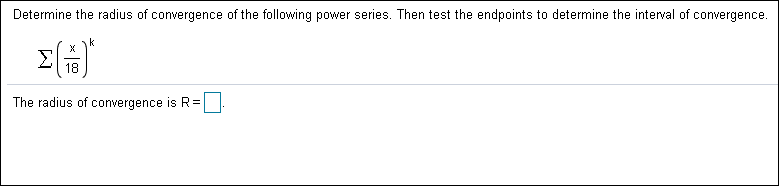# Determine the radius of convergence of the following power series. Then test the endpoints to determine the interval of convergence. k X 18 The radius of convergence is R

Questionhelp_outlineImage TranscriptioncloseDetermine the radius of convergence of the following power series. Then test the endpoints to determine the interval of convergence. k X 18 The radius of convergence is R fullscreen